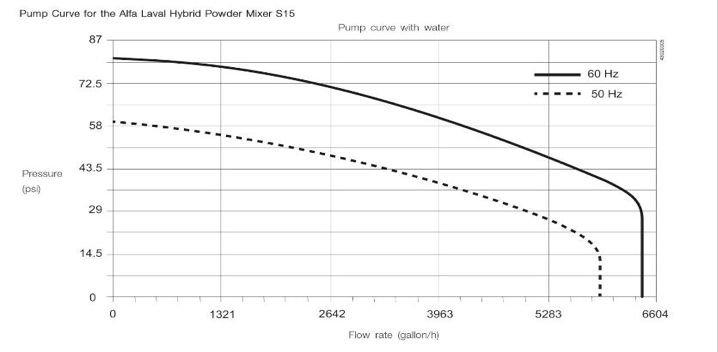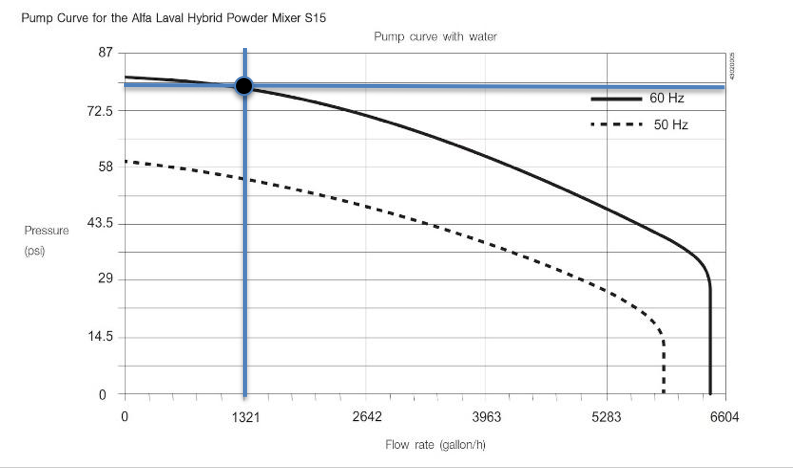How to Read a Pump CurvePump curves give you the information you need to determine a pump's ability to produce flow under the conditions that affect pump performance. Curves help you choose the right pump based on the application variables such as head (water pressure) and flow (the volume of liquid you have to move in a given time period).

For example, if you know the flow rate your application requires, you find the gallons per minute (or hour) rate along the bottom horizontal line of the curve and then draw a line up to the head/PSI you require. The curve will show you if the pump you have selected will perform in that application.

What is a Pump Curve?

Curves typically include performance metrics based on pressure, flow, horsepower, impeller trim, and Net Positive Suction Head Required (NPSHr).

Pump curves are useful because they show pump performance metrics based on head (pressure) produced by the pump and water-flow through the pump. Flow rates depend on pump speed, impeller diameter, and head.

Head is the height to which a pump can raise water straight up. Water creates pressure or resistance, at predictable rates, so we can calculate head as the differential pressure that a pump has to overcome in order to raise the water. Common units are feet of head and pounds per square inch. As Figure 1 illustrates, every 2.31 feet of head equals 1 PSI.

The Formula for PSI: Feet of Head/2.31 = PSI

Flow is the volume of water a pump can move at a given pressure. Flow is indicated on the horizontal axis in units like gallons per minute, or gallons per hour, as shown in Figure 2.Fig. 2. A basic pump curve shows a pump's performance range. In this curve, head is measured in PSI; flow is measured in gallons per hour.

While pump curves help you select the right pump for the job, you first have to know the total dynamic head for the application.

Total Dynamic Head (TDH) is the amount of head or pressure on the suction side of the pump (also called static lift), plus the total of 1) height that a fluid is to be pumped plus 2) friction loss caused by internal pipe roughness or corrosion.

TDH = Static Height + Static Lift + Friction Loss

Static Lift is the height the water will rise before arriving at the suction side of the pump.

Static Height is the maximum height reached by the pipe on the discharge side of the pump.

Friction Loss (or Head Loss) are the losses due to friction in the pipe at a given flow rate.

How to Use Performance Pump Curves in Selecting Equipment: The Basics

Let's say you want to know the flow rate you can achieve from the pump in Figure 3 at 60 Hz when the design pressure is 80 PSI. In this case, the curve shows that the pump can achieve a flow rate of 1321 gallons per hour at 80 PSI of discharge pressure.Fig. 3. The pump represented in this curve can generate 80 PSI of discharge pressure at a flow rate of 1321 gallons per hour.

Because some pumps operate across a range of horsepower, their curves will include additional information. Figure 4, for example, features a pump that can operate from 2 to 10 horsepower depending on desired performance.

For additional curves, see Alfa Laval LKH performance curves.

Impeller Trim Size

Impeller size is another variable for meeting performance requirements. The curve above shows impeller trim sizes, at the right end of each curve, ranging from a minimum of 4.33" to a maximum of 6.42".

Reducing impeller size enables you to limit the pump to specific performance requirements. The curve above shows maximum pump performance with a full-trim impeller, minimum pump performance with a minimum-trim impeller, and performance delivered by the design-trim impeller, or the impeller trim closest to the design condition. Impellers are typically trimmed 0.20 inches (or 5mm) at a time.

Impeller size is also a factor when handling shear sensitive liquids, or liquids that change viscosity when under pressure.

In addition to pressure and flow, the curve at the bottom of Figure 4 indicates NPSHr, which stands for Net Positive Suction Head Required. NPSHr is the minimum amount of pressure required on the suction side of the pump to avoid cavitation, or the introduction of air into the fluid stream. NPSHr is determined by the pump. You always want NPSHa>NPSHr.

NPSHa, with "a" standing for available, is determined by the process piping. You always want NPSHa to be greater that NPSHr. Without enough net positive suction, the pump will cavitate, which affects performance and pump life.

Efficiency and Performance Variables

Good pump efficiency means that a pump is not wasting energy in order to maintain its performance point. No pump is 100% efficient, however, in the work it has to do to transfer liquids.

When selecting a pump and motor combination, consider not only the total current demand but future demand to ensure your selection has the capacity to meet changing requirements. To that end, sizing the pump for performance variables rather than peak efficiency is a common practice.

For example, while the middle of the curve is generally where a pump is operating at maximum efficiency in terms of pressure and flow rate, moving right on the curve above shows an increase in horsepower needed to maintain a flow rate as head increases. For example, 2 hp is required for a flow rate of 40 gpm with 80 feet of head, but maintaining 40 gpm of flow at 110 feet of head would require a 3 hp motor.